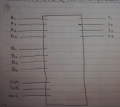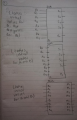# 4-BIT ALU PROTEUS

Joined Jul 1, 2020
2
Hello cool people, I'm new to Proteus and overall designing digital electronic circuits. I have to design a 4 BIT ALU unit capable of adding, subtracting, multiplying using full and half adders, so the information has to enter by 2 registers of 4 bits type D, and have a selector system that will decide if the ALU will add, subtract or multiply. Does anybody know how to do this? or give me guidance regarding it since I'm at level 0. Any help will be much appreciated. Thank you so much!!!

#### ericgibbs

Joined Jan 29, 2010
17,151
hi SK,
Welcome to AAC.
As this is an homework problem , please post your best attempt at answering, we can then help.
E

Joined Jul 1, 2020
2
Hi Eric! This is what I have in mind:

I’m thinking of first making a sub-circuit that has all the implementations needed (adding, subtracting, and multiplying). Like the picture I have drawn (P1).So what it will do is input the 2 registers, in this case A and B. Then select the type of operation to perform or to show in display (done by 0 or 1). Then make another sub-circuit with all the operations (adding, subtracting, and multiplying). As shown in the picture (P2).So then each one of the sub-circuit will have the internal circuit that makes them work. I have a full basic idea for it but don’t really know if it’s the right way to do it and how would I link all of it together.

I would really appreciate any guidance, thank you.

#### Irving

Joined Jan 30, 2016
3,311
It's a start. I'm assuming you have knowledge of basic logic gates and Boolean arithmetic and can write truth tables?

If not, this is going to be hard!

So you have the outline of the approach. I'd ignore multiply for now and focus on add & subtract functions. You have the idea of a subcircuit for each function and a selection mechanism for the function to be performed

So what do you think the next step will be?

#### Ian Rogers

Joined Dec 12, 2012
1,048
Most ALU's do shift left right rather than multiply I thought there was a ALU demo in the tutorials...

#### Irving

Joined Jan 30, 2016
3,311
yes, but let the OP get their head around the easy stuff and get thinking. No value in telling them the answer!